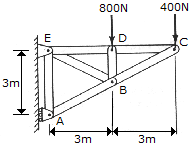# Engineering Mechanics - Structural Analysis - Discussion

### Discussion :: Structural Analysis - General Questions (Q.No.1)

1.Determine the force in each member of the truss and indicate whether the members are in tension or compression.

 [A]. CB = 447 N C, CD = 200 N T, DB = 800 N C, DE = 200 N T, BE = 447 N T, BA = 894 N C, AE = 800 N T [B]. CB = 447 N T, CD = 200 N C, DB = 800 N T, DE = 200 N C, BE = 447 N C, BA = 894 N T, AE = 800 N C [C]. CB = 894 N T, CD = 800 N C, DB = 800 N T, DE = 800 N C, BE = 894 N C, BA = 1789 N T, AE = 800 N C [D]. CB = 894 N C, CD = 800 N T, DB = 800 N C, DE = 800 N T, BE = 894 N T, BA = 1789 N C, AE = 800 N T

Explanation:

No answer description available for this question.

 Aravinth said: (Aug 8, 2011) Please give explaination also.

 Md Waseem said: (Aug 25, 2011) Please exlain us.

 Fahim said: (Feb 25, 2012) By using method of joints, i can get CB CD DB and DE, but when doing joint B i get the wrong answer. Can anyone help? Thanks in advance. (i know i have enough to answer the question, but i would like to be able to work through the whole thing)

 Prince Tetteh said: (Mar 20, 2015) Ok guys. First consider a free body diagram for the whole truss structure to find the reactions at supports E and A by applying the equations of equilibrium for its external forces. So taking summation of moment about E = 0, 3Ra-3(800)-6(400) = 0. Ra = 1600 N. Sum of horizontal forces = Re(x)-800-400 = 0; Re(x) = 1200 N. Sum of vertical forces = Re(y)-Ra = 0; Re(y) = 1600 N. Considering each member force to be a tension, each joint will be analyzed so taking joint C, Sum of horizontal forces = 400+F(CB) sin(26.57) = 0, F(CB) = -894 N T or F(CB) = 894 N C. Sum of vertical forces = -F(CB) cos (26.57)-F(CD) = 0; F(CD) = 800 N T. At joint D, F(DC) = F(DE) = 800 N T F(DB) = -800 N T = 800 N C. At joint E, Sum of vertical forces = F ED)+F(EB) sin(63.43)-Re(y) = 0. F(EB) = (Re(Y)-F(ED))/sin(63.43) = 894 N T. Sum of horizontal forces = F(EA) = F(EB) cos(63.43) = Re(x). F(EA) = 1200-894 cos(63.43) = 800 N T. At joint B, Sum of horizontal forces = 0. F(BA) cos(63.43)-F(BC) cos(63.43)-F(BE) cos(63.43)-F(BD) = 0. F (BA) = -1789 N T = 1789 N C.

 Mamdooh said: (Apr 5, 2016) Thank you, @Prince Tetteh. I will solve it in my assignment.

 Hasmukh Vasava said: (Sep 27, 2017) I don't understand how to arrive sin and cos (26.57) in summation of vertical forcer and sin and cos (63.43) in horizontal forces. Please help me to get it.

 Bakri said: (Apr 24, 2018) How do you end up with sum of horizontal forces as Re(x)-800-400? Please explain @Prince Tetteh.